# Set Partition Problems

This is my first post of 2019 and my first post in a while. There was one posted a few months ago, but not really geared towards the algorithms and learning focus of the site. I have been doing a lot of coding in my spare time, but honestly life has just gotten in the way. Its not a bad thing, but life is life and sometimes I have to prioritize the things. In particular, I have been having ideas and actually coding things up but the time it takes to clean up code, write a blog entry and finding a nice way to visualize these things has been something that I haven’t been able to really focus on as much as I’ve wanted to.

That said, I want to talk to you about the Set Partition Problem today. You can go to Wikipedia to get more information about this problem, but I will give you a brief introduction to it and then talk about two different approaches to it. The problem assumes that we are given as input a (multi)-set S. The reason we say it is a multi-set and not a simple set is because we can have the same element appear multiple times in the set. So if S1 = {2} and S2 = {2, 2}, then although as sets they are both equal to the set {2} = S1, as multi-sets allow for multiple instances of an element. The elements of S are assumed to be positive integers.

So given this multi-set S, we ask the question of can the elements of S be divided into two smaller multi-sets, C1 and C2 where

• C1 [union] C2 = S
• C1 [intersect] C2 = [empty set]
• [Sigma]_[x in C1] = [Sigma]_[x in C2].C1 [union] C2 = S, C1 [intersect] C2 = [empty set], and [Sigma]_[x in C1] = [Sigma]_[x in C2]

The first two bullets above say that the sets C1 and C2 form a partition of S. The third bullet says that the sums of the elements in the two children multi-sets are equal.

This problem is known to be NP Complete. This means that it is one of the more difficult decision problems. Because of this finding an algorithm that solves this problem exactly will generally take a long running time. And finding an algorithm that runs quickly will more than likely be incorrect in some instances.

I will show you two approaches to this problem. One is based on Dynamic Programming (DP) and one is based on Greedy Algorithms. The DP version solves the problem exactly but has a slow running time. The greedy algorithm is fast but is not guaranteed to always give the correct answer.

Dynamic Programming is based on the principle of optimality. This says that in order to have a correct solution to the overall problem, we need optimal solutions to all subproblems of this problem. This is done by keeping track of a table which can be used for looking up these subproblems and the optimal solutions to these subproblems.

For this problem, we will build a table where the rows represent the final sums and columns represent subsets of the given multi-set (containing the first 0…n elements). The question we are repeatedly asking is “can we find a subset of the set in this column whose sum is exactly the given rowsum?” Here is an example of the DP algorithm on the multi-set {5, 6, 5, 6, 7}.

Greedy Algorithms are generally based on sorting the elements based on some principle and using that to try to answer the underlying question. The main problem with this approach is that it is very short sided because they do not look at the overall picture. Such algorithms are known for finding local optima that are not always globally optimal. The benefit to these problems though is that they are generally easier to code and easier to understand.

For the Set Partition Problem , the Greedy approach is to sort elements in descending order. Once this is done, the goal is to keep two subsets while iterating through the array, adding the element to the smaller of the two sets whenever possible.

For more examples, check out Set Partition Problems

# Unidirectional TSP Puzzles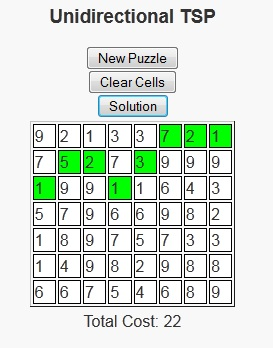As we’ve entered the late spring into early summer season, I’ve found myself wanting to go out more to sit and enjoy the weather. One of these days recently I sat in the park with a good book. On this occurrence, I decided not to go with a novel as I had just finished “Incarceron“, “The Archer’s Tale“, and “14 Stones” – all of which were good reads, but I felt like taking a break from the novels.

Just as a side note, 14 Stones is a free book available on smashwords.com and I’ve now read about 6 books from smashwords.com and haven’t been disappointed yet. My favorite is still probably “The Hero’s Chamber” because of the imagery of the book, but there are some well written ebooks available there by some good up and coming writers for a reasonable price, with some being free.

So with the desire to read, but not being in the mood for novels I decided to pick up one of my non-text but still educational books that make me think. This day it was “Programming Challenges“. I browsed through the book until I found one that I could lay back, look at the water, and think about how to solve it.

The programming puzzle the peaked my interest was called “Unidirectional TSP”. We are given a grid with m rows and n columns, with each cell showing the cost of using that cell. The user is allowed to begin in any cell in the first column and is asked to reach any cell in the last column using some minimum cost path. There is an additional constraint that once a cell is selected in a column, a cell in the next column can only be chosen from the row directly above, the same row, or the row directly below. There is a javascript version of this puzzle available here.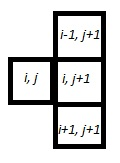Fundamentally, the problem is asking for a path of shortest length. Many shortest length problems have a greedy structure, but this one gained my interest because the greedy solution is not always optimal in this case. So I took a moment to figure out the strategy behind these problems. Once I had that solution, I decided that it would be a good program to write up as a puzzle.

In this puzzle version, users will click the cells they wish to travel in each column in which case they will turn green (clicking again will turn them white again). Once the user clicks on a cell in the last column, they will be notified of whether or not they have chosen the minimum path. Or if users are unable to solve a puzzle, the “Solution” button can be pressed to show the optimal path and its cost.

# Triangle Sum Puzzle

This is probably a consequence of being a mathematician, but I have always enjoyed number puzzles. I think that there is a general simplicity and universality in numbers that are not present in things like word puzzles, where the ability to reach a solution can be limited to the vocabulary of the user.

The fact that these are puzzles and not simply homework exercises also helps because we often find people sharing difficulties and successes stories over the water cooler or at the lunch table. The fact that many of these math puzzles can teach some of the same concepts as homework problems (in a more fun and inclusive way) is generally lost on the user as their primary interest is generally on solving the puzzle in front of them, or sometimes solving the more general form of the puzzle.

Today’s post is about a puzzle that was originally shared with me over a lunch table by a friend who thought it was an interesting problem and asked what I thought about it. I didn’t give the puzzle much further thought (he had correctly solved the puzzle) until I saw it again in “Algorithmic Puzzles” by Anany Levitin and Maria Levitin. It was then that I thought about the more general form of the puzzle, derived a solution for the problem, and decided to code it up as a script for my site.

Below is a link to the puzzle: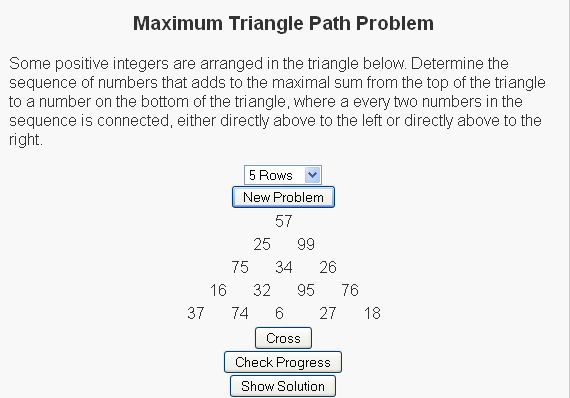We have a set of random numbers arranged in a triangle and the question is to find the path of maximum sum from the root node (the top node) to the base (one of the nodes on the bottom row) with the rules that
(1) Exactly one number must be selected from each row
(2) A number can only be selected from a row if (a) it is the root node or (b) one of the two nodes above it has been selected.

For the sample
So for the sample problem in the picture, the maximal path would go through nodes 57, 99, 34, 95, and 27.

For more of these puzzles check out the script I write here and be sure to let me know what you think.

# The Bridge Crossing Problem

Most puzzles are fun in their own right. Some puzzles are so fun that they have the added benefit that they are likely to come up in unexpected places, like maybe in a job interview. I was recently reading a paper by Günter Rote entitled “Crossing the Bridge at Night” where Rote analyzes such a puzzle. Upon finishing the paper, I decided to write a script so that users could see the general form of this puzzle.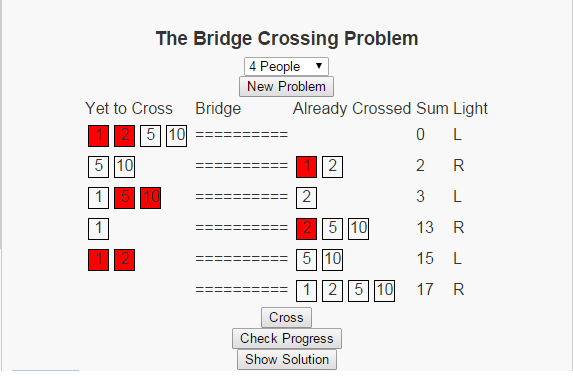The problem can be stated as follows: There is a set of people, lets make the set finite by saying that there are exactly n people, who wish to cross a bridge at night. There are a few restrictions that make crossing this bridge somewhat complicated.

• Each person has a travel time across the bridge.
• No more than two people can cross the bridge at one time.
• If two people are on the bridge together, they must travel at the pace of the slower person.
• There is only one flashlight and no party (of one or two people) can travel across the bridge without the flashlight.
• The flashlight cannot be thrown across the bridge, and nobody can go to the store to purchase another flashlight

The image above shows the optimal solution when the 4 people have travel times of 1, 2, 5, and 10. The script I have written allows users to work with different numbers of people with random travel times. Give it a try and see if you can spot the patterns in the solution.

# The A* Algorithm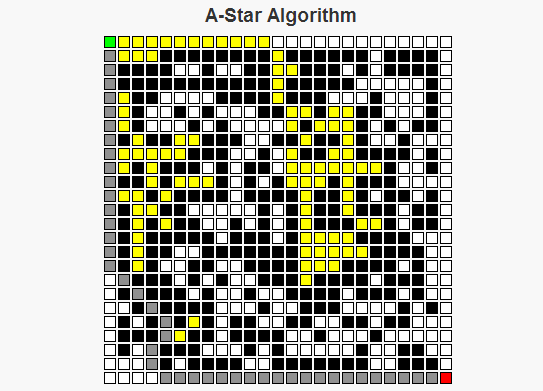As a child I remember traveling on road trips, sitting in the back of a car trying to do my best to keep myself busy for what would occupy the next six to ten hours of my life. One of the things I grew to like were the simple maze books that were sold on the magazine racks at some of the gas stations where we’d stop for food. There are two basic strategies I employed for solving these mazes: For simpler mazes, I could generally just take a look at the overall maze structure, decide upon a path through the maze, then write a path without any mistakes. For more complex mazes though, I would generally begin a route that looks the most promising. If that route reaches a point where I can see that it will be impossible to finish, then I’d go back to where the decision was made, exclude the route I had just tried, and select the “most promising” remaining route. I’d continue this process until I had completed the maze, or until it became simple enough for me to solve the maze using only my memory.

The A* Algorithm works in a similar manner to the second approach I just described. We begin at a starting point, and consider where to move next from that starting point. The set of possible options for this next move is determined by the neighbor function for a given cell. For each neighbor the algorithm estimates the length of the route through that cell by calculating the sum of two values, f(n) = g(n) + h(n), where

g(n) is the (known) cost to travel from the starting point to the cell n.
h(n) is the (approximate) cost to travel from the cell n to the final cell.

The sum g(n) + h(n) allows us to approximate the total cost of a route through the cell n.

The A* algorithm begins at the starting position of the maze. There are two sets we will be considering throughout the process of determining the optimal route, called the closedSet and the openSet. The elements of closedSet are the nodes whose total distance from the starting position has been calculated, whereas the elements of openSet represents nodes whose total distance is still under consideration. There is also a map called prev which is used to reconstruct the path. Below is how the algorithm operates:

A* Algorithm Pseudocode
closedSet is the empty set
openSet = {start}
prev is the empty set

While there are still elements in openSet,
Find the element c* in openSet with the lowest value f(c).
If c* is the target position
Reconstruct the path.
Else If c* is not the target position,
Remove c* from openSet
Add c* to closedSet
For each neighbor n of c* that is not in closedSet,
Calculate a temporary g value, temp_g(n) = g(c*) + dist(c*, n).
If n is not in openSet, or if n is in openSet and temp_g(n) < g(n),                     Set prev(n) = c*                     Set g(n) = temp_g(n)                     Set f(n) = g(n) + h(n).                     if n is not in openSet                          add n to openSet.      End If End While End Algorithm An important question becomes what makes a good heuristic function to approximate the distance to the goal. This can lead to an in depth discussion based on the word "good", but the necessary condition for any heuristic is that it NEVER over-estimates the cost of the path from the cell to the goal. Some examples of possible heuristics for mazes are the Euclidean Distance (the square root of the sum of the squares of the horizontal and vertical differences in distances, i.e. dE(x, y) =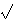(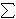i(xi – yi)2) and TaxiCab Distance (the sum of the differences in the horizontal and vertical dimensions, i.e. dT(x, y) =i|xi – yi|). Both of these are feasible metrics for heuristics on a maze. Other herusitics, like h(n) = 0 for all n are possible, but doing this would make the algorithm treat all cells equally and ignore the heuristic part of the A* algorithm, turning it into Dijkstra’s algorithm.

I’ve written a script that generates random mazes and uses the A* algorithm to find the optimal path through this maze. For this script, I used the taxicab distance heuristic.

Check it out and let me know what you think.

# Interactive Tutorial of Kruskal’s AlgorithmI just finished working on another implementation of Kruskal’s algorithm. This version depends more on user input to generate the minimum spanning path tree.

Check it out.

# Interactive Tutorial of Prim’s AlgorithmI just finished working on another implementation of Prim’s algorithm. This version depends more on user input to generate the minimum spanning path tree.

Check it out.

# Approximating the Set Cover ProblemI just finished my weekly task of shopping for groceries. This can be a somewhat daunting task because I generally have a list of things that I’ll need which cannot all be purchased at a single location. What often happens is that I find that many of the items on my list are ONLY offered at certain stores – generic brands of certain items for example. My goal then changes from minimizing the total amount of money spent to minimizing the number of stores that I must visit to purchase all of my items.

To formulate this as a mathematical problem, suppose that I have a grocery list of items I would like to buy, represented by the lists item1, item2, …, itemn, where n represents the number of items I have on this list. Suppose also that there are stores Store1, Store2, …, Storem (each one distinct) that offer some combination of items I have on my list. What I would like to do is minimize the number of stores I have to visit to purchase these items.

The problem I just described is famous because it is one that many people face on a regular basis. In a more general form, it is so famous that it has a name for it, called the Set Cover Problem (or the Minimum Set Cover Problem). In the general form of this problem, we replace the grocery list with a set of items called our universe. The lists of items offered at each store are the collections of subsets of the universe. In the problem, as in the example above, we would like to select enough subsets from this collection that we are able to obtain every element in our universe. We would like to do this with as low a number of sets as possible.

In my previous post, I described the 21 problems that Karp proved were NP-Complete. Set Cover was one of those problems, showing that this is a hard problem to solve. What I will do is introduce three ways to reach a near-optimal solution relatively quickly.

Greedy Method

One of the first approaches one may take to solve this problem is to repeatedly select the subset that contains the most new items. That’s how the greedy approach to set cover operates. The method knows to terminate when all elements belong to one of the selected sets. In the shopping example above, this would be accomplished by visiting the store that had the most items on my list and purchasing those items at this store. Once this is done, the items that have been purchased can be crossed off my list and we can visit the store with the most items on my remaining list, stopping when the list is empty.

Linear Programming Relaxation

Instead of stating the set cover problem with words, there is a way of describing the situation with mathematical inequalities. For instance, suppose that the soap I like to purchase is only available at stores Store1, Store4 and Store9. Then I could introduce a variable xi for each store i and the requirement that I purchase this soap can be restated as :

x1 + x4 + x9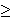1

Because we can either purchase some items or not purchase these items, each variable xi is 0 or 1 (called a binary variable). We can introduce similar constraints for each element in our universe (or on our grocery list). These inequalities (called constraints) have the form:

for each element e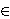U,i | eSi xi1

Our goal of minimizing the number of sets chosen (stores visited) can be stated by the objective function:
minimize1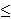in xi

So the mathematical formulation for this problem can be stated as

minimize1in xi
Subject to
for each element eU,i | eSi xi1
for each set i, xi{0, 1}.

Formulations of this type, where variables are restricted to a finite set (in this case the x variables being either 0 or 1) are called integer programs. Unfortunately, there is no easy way to solve these formulations either. However, there is a related problem which can be solved quickly.

Instead of restricting the x variables to the values of 0 or 1, we could allow them to take on any value within this range, i.e. 0xi1 for each set Si. Doing this converts the problem from an integer programming problem into a linear programming problem (called the LP-Relaxation), which can be solved quickly. The issue with this method though is that the solution obtained by an LP-Relaxation is not guaranteed to be an integer. In this case, how do we interpret the values xi?

Randomized Rounding Method

One approach to dealing with a non-integer solution to the LP-Relaxation is to treat the xi values as probabilities. We can say that xi is the probability that we select set i. This works because each value of xi is in the range of 0 to 1, which is necessary for a probability. We need to repeatedly select sets with their associated probabilities until all elements in our universe are covered. Selecting our sets based on this procedure is the randomized rounding approach.

Deterministic Rounding Method

A second approach to dealing with a non-integer solution to the LP-Relaxation is to base our solution on the most occurring element. If we let f be this frequency (i.e.the number of sets that the most occurring element occurs in), then we can define a solution by selecting set i if the LP=Relaxation solution gives the variable xi a value of at least (1/f).

None of these three approaches is guaranteed to give an optimal solution to an instance of this problem. I will not go into it in this post, but these can all be shown to be within some guaranteed range of the optimal solution, thus making them approximation algorithms.

Hope you enjoy.

# Knapsack ProblemsTo help understand this problem, I want you to think about a common situation in many people’s lives. You have a road trip coming up today and you’ve overslept and are at risk of missing your flight. And to top matters off, you were planning to pack this morning but now do not have the time. You quickly get up and begin to get ready. You grab the first bag you see and quickly try to make decisions on which items to take. In your head you’re trying to perform calculations on things you’ll need for the trip versus things that you can purchase when you get there; things that you need to be able to have a good time versus things you can do without. And to top matters off, you don’t have time to look for your ideal luggage to pack these things. So you have the additional constraint that the items you pick must all fit into this first bag you found this morning.

The situation I described above is a common problem. Even if we ignore the part about the flight, and just concentrate on the problem of trying to put the most valuable set of items in our bag, where each item has its own value and its own size limitations, this is a problem that comes up quite often. The problem is known (in the math, computer science and operations research communities) as the knapsack problem. It is known to be difficult to solve (it is said to be NP-Hard and belongs to a set of problems that are thought to be the most difficult problems within its class). Because of this difficulty, this problem has been well studied.

What I provide in my script are two approaches to solving this problem. The first is a greedy approach, which selects a set of items by iteratively choosing the item with the highest remaining value to size ratio. This approach solves very fast, but can be shown to give sub-optimal solutions.

The second approach is a dynamic programming approach. This algorithm will solves the problem by ordering the items 0, 1, …, n and understanding that in order to have the optimal solution on the first i items, the optimal solution must have been first selected on the fist i-1 items. This algorithm will optimally solve the problem, but it requires the computation of many sub-problems which causes it to run slowly.

Update (4/2/2013): I enjoy this problem so much that I decided to implement two additional approaches to the problem: Linear Programming and Backtracking.

The Linear Programming approach to this problem comes from the understanding that the knapsack problem (as well as any other NP-Complete problem) can be formulated as an Integer Program (this is a mathematical formulation where we seek to maximize a linear objective function subject to a set of linear inequality constraints with the condition that the variables take on integer values). In the instance of the knapsack problem we would introduce a variable xi for each item i; the objective function would be to maximize the total value of items selected. This can be expressed as a linear objective function by taking the sum of the products of the values of each item vi and the variable xi; the only constraint would be the constraint saying that all items fit into the knapsack. This can be expressed as a linear inequality constraint by taking the sum of the products of the weights of each item wi and the variable xi. This sum can be at most the total size of the knapsack. In the integer programming formulation, we either select an item or we do not. This is represented in our formulation by allowing the variable xi = 1 if the item is selected, 0 otherwise.

The LP relaxation of an integer program can be found by dropping the requirements that the variables be integer and replacing them with linear equations. So in the case of the knapsack problem, instead of allowing the variables to only take on values of 0 and 1, we would allow the variables to take on any value in the range of 0 and 1, i.e 0 <= xi <= 1 for each item i. We can then solve this LP to optimality to get a feasible solution to the knapsack problem. The second knapsack approach I implemented today is through backtracking. Similar to the Dynamic Programming approach to this problem, the backtracking approach will find an optimal solution to the problem, but these solutions generally take a long time to compute and are considered computationally inefficient. The algorithm I implemented here first orders the item by their index, then considers the following sub-problems for each item i "What is the best solution I can obtain with this initial solution?". To answer this question, the algorithm begins with an initial solution (initially, the empty set) and a set of unchecked items (initially, all items) and recursively calls itself on sub-problems with an additional item as a part of the initial solution and with this item removed from the unchecked items. So check out my knapsack problem page. I think its a good way to be introduced to the problem itself, as well as some of the techniques that are used in the fields of mathematics, computer science, engineering and operations research.

Other Blogs covering this topic:
Journey to the Four Point Oh

# Dijkstra’s Algorithm

I’ve just written a script that executes Dijkstra’s algorithm that seeks to find the shortest path tree on a randomly generated graph.

Given a weighted graph G and a vertex s, the single node shortest path problem seeks to find the shortest path from s to every other vertex in this graph such that the sum of the weights of the edges along these paths is minimized. One famous algorithm for this problem is Dijkstra’s Algorithm, which constructs this shortest path tree using techniques of greedy algorithms and dynamic programming.

Dijkstra’s Algorithm works as follows.

• Initially we have an empty path tree T, and assume that the distance to every vertex in the graph has some maximum cost, say infinity, i.e. w(v) = infinity for all v in V.
• Add the node s to the tree, and give the associated path cost a value of zero, i.e. w(s) = 0.
• Find the edge which is adjacent to T that adds the vertex whose cost is minimum, i.e. we look for an e = (u, v) such that u is in T, and v is not in T and w(u) + cost(u, v) < w(v) is minimum. If no such edge exists go to 5.
• Add the corresponding edge and vertex to the tree, and update the weight vector of the vertex v. Go to 3.
• The path tree T now represents the shortest path from the vertex s to all other vertices reachable in the graph G. The weight vector w represents the costs of these paths.

For an example of Dijkstra’s algorithm executed on the Graph with the following adjacency matrix:

 0 1 2 3 4 5 6 7 0 – – 2 4 – – 20 – 1 – – – 11 11 7 5 – 2 2 – – – 10 – 7 – 3 4 11 – – – – – 2 4 – 11 10 – – – – – 5 – 7 – – – – – 14 6 20 5 7 – – – – – 7 – – – 2 – 14 – –Suppose we are interested in discovering the shortest paths from the node 0.

Initially Dijkstra’s algorithm constructs an empty path tree, T = {}.

Because we want to discover the shortest paths from the node 0, our first step will be to add this node to the tree and update the weight vector.
T = {0}
w(0) = 0.

Now we will consider the edges adjacent to T, {(0, 2), (0, 3), and (0, 6)}. Our assumption is that the shortest path of every vertex in T has already been computed correctly and we will seek the edge that minimizes the value w(u) + c(u, v), where u is a member of T and (u, v) is an edge adjacent to T. The edge that does that currently is (0, 2) since w(0) = 0 and c(0, 2) = 2. We add the node 2 to T and update the weight vector.
T = {0, 2}
w(2) = 2.

The edges adjacent to T are now {(0, 3), (0, 6), (2, 4), (2, 6)}.
The associated path costs are:
w(0) + c(0, 3) = 0 + 4 = 4
w(0) + c(0, 6) = 0 + 20 = 20
w(2) + c(2, 4) = 2 + 10 = 12
w(2) + c(2, 6) = 2 + 7 = 9
We can see that the edge that minimizes the value w(u) + c(u, v), where u is a member of T and (u, v) is an edge adjacent to T is (0, 3). We add the node 3 to T and update the weight vector.
T = {0, 2, 3}
w(3) = 4

The edges adjacent to T are now {(0, 6), (2, 4), (2, 6), (3, 1), (3, 7)}.
The associated path costs are:
w(0) + c(0, 6) = 0 + 20 = 20
w(2) + c(2, 4) = 2 + 10 = 12
w(2) + c(2, 6) = 2 + 7 = 9
w(3) + c(3, 1) = 4 + 11 = 15
w(3) + c(3, 7) = 4 + 2 = 6
We can see that the edge that minimizes the value w(u) + c(u, v), where u is a member of T and (u, v) is an edge adjacent to T is (3, 7). We add the node 7 to T and update the weight vector.
T = {0, 2, 3, 7}
w(7) = 6

The edges adjacent to T are now {(0, 6), (2, 4), (2, 6), (3, 1), (5, 7)}.
The associated path costs are:
w(0) + c(0, 6) = 0 + 20 = 20
w(2) + c(2, 4) = 2 + 10 = 12
w(2) + c(2, 6) = 2 + 7 = 9
w(3) + c(3, 1) = 4 + 11 = 15
w(7) + c(5, 7) = 6 + 14 = 20
We can see that the edge that minimizes the value w(u) + c(u, v), where u is a member of T and (u, v) is an edge adjacent to T is (2, 6). We add the node 6 to T and update the weight vector.
T = {0, 2, 3, 6, 7}
w(6) = 9

The edges adjacent to T are now {(2, 4), (3, 1), (5, 7) (6, 1)}.
The associated path costs are:
w(2) + c(2, 4) = 2 + 10 = 12
w(3) + c(3, 1) = 4 + 11 = 15
w(7) + c(5, 7) = 6 + 14 = 20
w(6) + c(6, 1) = 9 + 5 = 14
We can see that the edge that minimizes the value w(u) + c(u, v), where u is a member of T and (u, v) is an edge adjacent to T is (2, 4). We add the node 4 to T and update the weight vector.
T = {0, 2, 3, 4, 6, 7}
w(4) = 12

The edges adjacent to T are now {(3, 1), (5, 7), (6, 1), (4, 1)}.
The associated path costs are:
w(3) + c(3, 1) = 4 + 11 = 15
w(7) + c(5, 7) = 6 + 14 = 20
w(6) + c(6, 1) = 9 + 5 = 14
w(4) + c(4, 1) = 12 + 11 = 23
We can see that the edge that minimizes the value w(u) + c(u, v), where u is a member of T and (u, v) is an edge adjacent to T is (6, 1). We add the node 1 to T and update the weight vector.
T = {0, 1, 2, 3, 4, 6, 7}
w(1) = 14

The edges adjacent to T are now {(5, 7), (1, 5)}.
The associated path costs are:
w(7) + c(5, 7) = 6 + 14 = 20
w(1) + c(1, 5) = 14 + 7 = 21
We can see that the edge that minimizes the value w(u) + c(u, v), where u is a member of T and (u, v) is an edge adjacent to T is (5, 7). We add the node 5 to T and update the weight vector.
T = {0, 1, 2, 3, 4, 5, 6, 7}
w(5) = 20

Now that T contains all the nodes from the tree, we know the shortest path from node 0 to all other nodes and and have solved the problem. The associated weight vector is w = [0, 14, 2, 4, 12, 20, 9, 6].

To learn more and see more examples, view Dijkstra’s Algorithm at LEARNINGlover.com